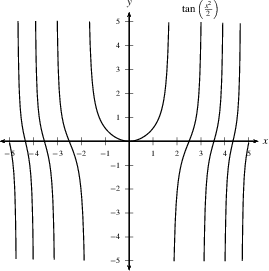Home

#### The Fundamental Theorem of Calculus

Three Different Quantities
The Whole as Sum of Partial Changes
The Indefinite Integral as Antiderivative
The FTC and the Chain Rule

#### The Indefinite Integral and the Net Change

Indefinite Integrals and Anti-derivatives
A Table of Common Anti-derivatives
The Net Change Theorem
The NCT and Public Policy

#### Substitution

Substitution for Indefinite Integrals
Revised Table of Integrals
Substitution for Definite Integrals

#### Area Between Curves

The Slice and Dice Principle
To Compute a Bulk Quantity
The Area Between Two Curves
Horizontal Slicing
Summary

#### Volumes

Slicing and Dicing Solids
Solids of Revolution 1: Disks
Solids of Revolution 2: Washers
Volumes Rotating About the $y$-axis

Behind IBP
Examples
Going in Circles

#### Integrals of Trig Functions

Basic Trig Functions
Product of Sines and Cosines (1)
Product of Sines and Cosines (2)
Product of Secants and Tangents
Other Cases

#### Trig Substitutions

How it works
Examples
Completing the Square

#### Partial Fractions

Introduction
Linear Factors
Improper Rational Functions and Long Division
Summary

#### Strategies of Integration

Substitution
Integration by Parts
Trig Integrals
Trig Substitutions
Partial Fractions

#### Improper Integrals

Type I Integrals
Type II Integrals
Comparison Tests for Convergence

#### Differential Equations

Introduction
Separable Equations
Mixing and Dilution

#### Models of Growth

Exponential Growth and Decay
Logistic Growth

#### Infinite Sequences

Close is Good Enough (revisited)
Examples
Limit Laws for Sequences
Monotonic Convergence

#### Infinite Series

Introduction
Geometric Series
Limit Laws for Series
Telescoping Sums and the FTC

#### Integral Test

The Integral Test
When the Integral Diverges
When the Integral Converges

#### Comparison Tests

The Basic Comparison Test
The Limit Comparison Test

#### Convergence of Series with Negative Terms

Introduction
Alternating Series and the AS Test
Absolute Convergence
Rearrangements

The Ratio Test
The Root Test
Examples

#### Strategies for testing Series

List of Major Convergence Tests
Examples

#### Power Series

Finding the Interval of Convergence
Other Power Series

#### Representing Functions as Power Series

Functions as Power Series
Derivatives and Integrals of Power Series
Applications and Examples

#### Taylor and Maclaurin Series

The Formula for Taylor Series
Taylor Series for Common Functions
Adding, Multiplying, and Dividing Power Series
Miscellaneous Useful Facts

#### Applications of Taylor Polynomials

What are Taylor Polynomials?
How Accurate are Taylor Polynomials?
What can go Wrong?
Other Uses of Taylor Polynomials

#### Partial Derivatives

Definitions and Rules
The Geometry of Partial Derivatives
Higher Order Derivatives
Differentials and Taylor Expansions

#### Multiple Integrals

Background
What is a Double Integral?
Volumes as Double Integrals

#### Iterated Integrals over Rectangles

One Variable at the Time
Fubini's Theorem
Notation and Order

#### Double Integrals over General Regions

Type I and Type II regions
Examples
Order of Integration
Area and Volume Revisited

### Separable Equations

The next simplest case is when the rate of change $\frac{dy}{dx}$ can be factored in a way that separates the dependance of $x$ and $y$. In particular,

 A differential equation is called separable if it's of the form $$\frac{dy}{dx}=f(x)g(y).$$

If $g\ne 0$, we can cross-multiply to get $$\frac{dy}{g(y)} = f(x)\, dx$$ and then integrate both sides. This yields $$G(y) = F(x) + C,$$ where $F$ and $G$ are anti-derivatives of $f$ and $1/g$, respectively. As always, we need to plug in the value of one point to determine $C$.

 Example: Find the solutions of $$\frac{dy}{dx}=xy^2+x.$$ Solution: The equation is separable, since the rate of change $\frac{dy}{dx}$ can be written as $f(x)g(y)=x(y^2+1)$. Notice that $y^2+1\ne0$. Hence, $$\frac{dy}{y^2+1}=x\,dx$$ We integrate both sides $$\int\frac{dy}{y^2+1}=\int x\,dx$$ to get $$\arctan(y)=\frac{x^2}{2}+C,$$ or, $$y=\tan\left(\frac{x^2}{2}+C\right).$$